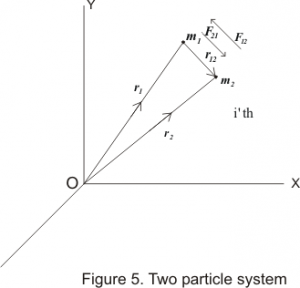# Two particle system and reduced mass

This article is about Two particle system and reduced mass. This topic comes under the chapter Dynamics of System of Particles. It is for B.Sc. students and comes under subject mechanics. For full chapter notes links
Dynamics of System of Particles

## Two particle system and reduced mass

• Two body problems with central forces can always be reduced to the form of one body problems.
• Consider a system made up of two particles. For an observer in any inertial frame of refrence relative motion of these two particles can be represented by the motion of a fictitious particle.
• The mass of this fictetious particle is known as the reduced mass of two particle system.
• Consider a system of two particles of mass m1 and m2 respectively. Let O be the origin of any inertial frame of reference and r1 and r2 be the position vectors of these particles at any time t w.r.t. origin O as shown bellow in the figure.• If no external force is acting on the system then the force acting on the system would be equal to mutual interaction between two particles. Let the force acting on m1 due to m2 be F21 and force acting on m2 due to m1 be F12 then equation of motion for particles m1 and m2 would be
\begin{equation}
{{\rm{F}}_{21}} = {m_1}\frac{{{d^2}{{\rm{r}}_1}}}{{d{t^2}}}
\end{equation}
and,
\begin{equation}
{{\rm{F}}_{12}} = {m_2}\frac{{{d^2}{{\rm{r}}_2}}}{{d{t^2}}}
\end{equation}
from 1 and 2
\begin{equation}
\frac{{{d^2}{{\rm{r}}_1}}}{{d{t^2}}} = \frac{{{{\rm{F}}_{21}}}}{{{m_1}}}
\end{equation}
and
\begin{equation}
\frac{{{d^2}{{\rm{r}}_2}}}{{d{t^2}}} = \frac{{{{\rm{F}}_{12}}}}{{{m_2}}}
\end{equation}
From the figure,
\begin{equation}
{{\rm{r}}_{12}} = {{\rm{r}}_{\rm{2}}} – {{\rm{r}}_1}
\end{equation}
so,
\begin{equation}
\frac{{{d^2}{{\rm{r}}_{12}}}}{{d{t^2}}} = \frac{{{d^2}{{\rm{r}}_2}}}{{d{t^2}}} – \frac{{{d^2}{{\rm{r}}_1}}}{{d{t^2}}}
\end{equation}
putting 3 and 4 in 6 we get
\begin{equation}
\frac{{{d^2}{{\rm{r}}_{12}}}}{{d{t^2}}} = \frac{{{{\rm{F}}_{12}}}}{{{m_2}}} – \frac{{{{\rm{F}}_{21}}}}{{{m_1}}}
\end{equation}
but from Newton’s first law of motion we have
F21 = –F12
then from equation 7 we have
$$\frac{{{d^2}{{\rm{r}}_{12}}}}{{d{t^2}}} = \frac{{{{\rm{F}}_{12}}}}{{{m_2}}} + \frac{{{{\rm{F}}_{12}}}}{{{m_1}}} = {{\rm{F}}_{12}}\left( {\frac{1}{{{m_1}}} + \frac{1}{{{m_2}}}} \right)$$
or,
$$\frac{{{d^2}{{\rm{r}}_{12}}}}{{d{t^2}}} = {{\rm{F}}_{12}}\left( {\frac{{{m_1} + {m_2}}}{{{m_1}{m_2}}}} \right)$$
$${{\rm{F}}_{12}} = \left( {\frac{{{m_1}{m_2}}}{{{m_1} + {m_2}}}} \right)\frac{{{d^2}{{\rm{r}}_{12}}}}{{d{t^2}}}$$
or,
\begin{equation}
\rm{F}}_{12}} = \mu \frac{{{d^2}{{\rm{r}}_{12}}}}{{d{t^2}}}
\end{equation}
where,
$$\mu = \left( {\frac{{{m_1}{m_2}}}{{{m_1} + {m_2}}}} \right)$$
is known as reduced mass of the system.
• This equation 8 represents a one body problem , because it is similar to the equation of motion of single particle of mass μ at a vector distance r12 from one of thr two particles, considered as the fixed centre of force.
• Thus original problem involving two particle system has now been reduced to that of one particle system which is easier to solve then original two body problem.
Case 1. m1 << m2
If the mass of any one particle in two particle system is very very less in comparison to other particle like in earth-satellite system then reduced mass of the system would be
\begin{equation*}
\mu = \left( {\frac{{{m_1}{m_2}}}{{{m_1} + {m_2}}}} \right) = \left( {\frac{{{m_1}}}{{1 + ({m_1}/{m_2})}}} \right)
\end{equation*}
or,
\begin{equation*}
\mu \approx \left( {1 – \frac{{{m_1}}}{{{m_2}}}} \right){m_1}
\end{equation*}
or, $\mu \approx {m_1}$
So the reduced mass of the two particle system would be equal to the particle having lesser mass.
• Case 2. m1 = m2 = m
• If the masses o8 the particles of a two particle system are same then
$$\mu = \left( {\frac{{{m_1}{m_2}}}{{{m_1} + {m_2}}}} \right) = \frac{{{m^2}}}{{2m}} = \frac{m}{2}$$
Hence reduced mass of the system would be equal to the one half of the mass of a single particle.

#### Recommended texts on Dynamics of system of particles

http://www.astro.uwo.ca/~houde/courses/PDF%20files/physics350/Systems_Particles.pdf

http://teacher.pas.rochester.edu/phy121/lecturenotes/Chapter09/Chapter9.html

http://www.spumone.org/courses/dynamics-notes/sysparticles/

https://ocw.mit.edu/courses/mechanical-engineering/2-003j-dynamics-and-control-i-spring-2007/lecture-notes/lec05.pdf